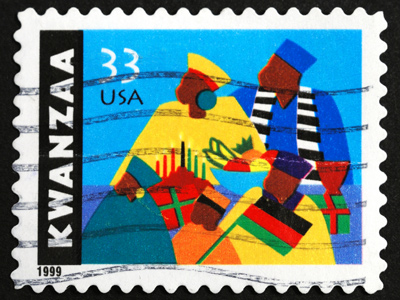33 is the biggest number you can divide into both the numerator and denominator of 33/66.

# Level 3-4 Numbers - Fractions - Simplification

Fractions of numbers are an important part of KS3 Maths. Fractions can be written more than one way. For instance, ten twentieths could be written as 1020. But 1020 has the same value as 24 or 12. Writing fractions in simpler forms like this is called simplification.

Simplification of a fraction means looking for the highest common factor on the top and bottom of it. Using the above example, in 1020 the highest common factor of 10 and 20 is 10. Divide each of the numbers by 10 and you get 1 and 2. So, in its simplest form 1020 is 12. Remember to look for the highest common factors in fractions and you'll soon find simplification is simple!

Practise is the best way to familiarise yourself with something. So, by the time you've finished this quiz, you'll be a dab hand! Take your time, read each question carefully and consider your answers. And don't forget the helpful comments after each question. They can help to explain things and make them a little clearer.

1.
What is the biggest number you can divide into both the numerator and denominator of 33/66?
3
6
11
33
Although both numbers are divisible by 11, it isn't the HIGHEST common factor
2.
What is the simplest way of writing 1/2?
1/4
1/3
1/2
1/5
1/2 is in its simplest form already!
3.
What is the biggest number you can divide into both the numerator and denominator of 71/92?
1/9
7/9
7/2
It's not possible
There is no common factor!
4.
How would you write 12/18 in its simplest form?
1/3
2/3
3/4
5/10
Divide 12 and 18 by 6 to find the answer
5.
How would you write 33/66 in its simplest form?
33/66
3/6
1/2
3/4
'Simplest form' is also known as 'lowest terms'
6.
What is the biggest number you can divide into both the numerator and denominator of 30/40?
3
4
10
There isn't one
Dividing 30 (the numerator) and 40 (the denominator) by 10 gives us 3/4
7.
How would you write 10/100 in its simplest form?
1/10
1/100
100/10
10/1
The common factor is 10
8.
What is 30/40 as a fraction in its simplest form?
1/2
3/4
30/40
300/400
The highest common factor of 30 and 40 is 10
9.
How would you write 9/81 in its lowest terms?
1/9
9/1
9/8
It's not possible
Both numbers are divisible by 9
10.
What is the biggest number you can divide into both the numerator and denominator of 12/18?
3
6
9
12
12 and 18 share a few factors - 1, 2, 3, 6 - but 6 is the highest